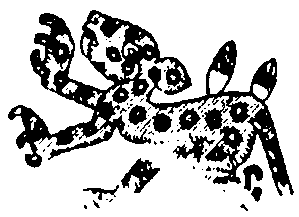# Event of the DayOne must know how to choose with exactitude the hour of the day or night so as to

successfully realise any activity of life.

An hour, a day, a month, and a year can be chosen for particular things. We do not enter

here into conventionality, for example, one should not use 21:00 hours, but rather 9:00

hours.

1.- EXAMPLE: Victor Manuel Chavez:

Date of Birth: 17-11-1921

Inner Urgency: 5. Fundamental Tendency: 5

Date to investigate: 14 May 1969. Time of event: 9 a.m

Day 14 = 1 + 4 = 5

Month 5

Year 1969 = 1 + 9 + 6 + 9 = 25 => 2 + 5 = 7

Day 5

Month 5

Year 7

17 = 1 + 7 = 8

8 + 5 (Fundamental Tendency) = 13

13 = 1 + 3 = 4 => Tendency of the Day, 14-5-1969

With the 4 one must know how to balance dealings, the details of any activity.

The most interesting hour of that day was at 9 o’clock in the morning.

4 (Tendency of the Day) + 9 (Hour of the day) = 13

13 = 1 + 3 = 4

He, at that hour, had to deal with a matter in his work, and it worked out well.

The 4 is the base, knows how to tally matters.

2.- EXAMPLE: Mrs Margarita Sancho Fernandez

Date of Birth: 4 November, 1943

Inner Urgency: 5 Fundamental Tendency: 8

Date to investigate: 13 June, 1969.

Day 13 = 1 + 3 = 4

Month 6

Year 1969 = 1 + 9 + 6 + 9 = 25 = 2 + 5 = 7

Day 4

Month 6

Year 7

17=1 + 7 = 8

8+8 (Fundamental Tendency) =16

1 + 6 = 7: Tendency of the Day 13-6-1969.

One must struggle so as not to fall, the energy must be intelligently directed, one must

struggle to raise oneself. The 16 is the Fulminated Tower.

The Event of the day is at 11 o’clock at night. 11 = 1 + 1 = 2.

7 (Tendency of the Day) +2 (hour of the day) = 9. 9: Event of the Day.

Her Event of the Day is to work with the Ninth Sphere.